Courses
Courses for Kids
Free study material
Free LIVE classes
More

# Maths Vocabulary for 2nd Grade Students## Vocabulary for 2nd Grade Students Maths

Level up in mathematics by learning some new useful words, specifically for second grade students, with our maths vocabulary! This maths glossary lesson contains 10 common words that are often taught in 2nd grade maths classes. The words that you will study in this article will be useful for you in all the future maths lessons as you will progress through the classes.

So, let’s get started with maths vocabulary second grade lesson!

## Maths Words for Second Grade

Here are some common maths words according to different maths topics:

### Number System

• Even Numbers - Numbers which leave remainder 0 when divided by 2. For example, 2, 4, 18, 22, etc.

• Odd Numbers - Numbers which when divided by 2 always leave the remainder “1”. For example, 3, 41, 67, 85, etc.

• Place Value - Every digit of a number has a certain value based on its position, which is called its place value.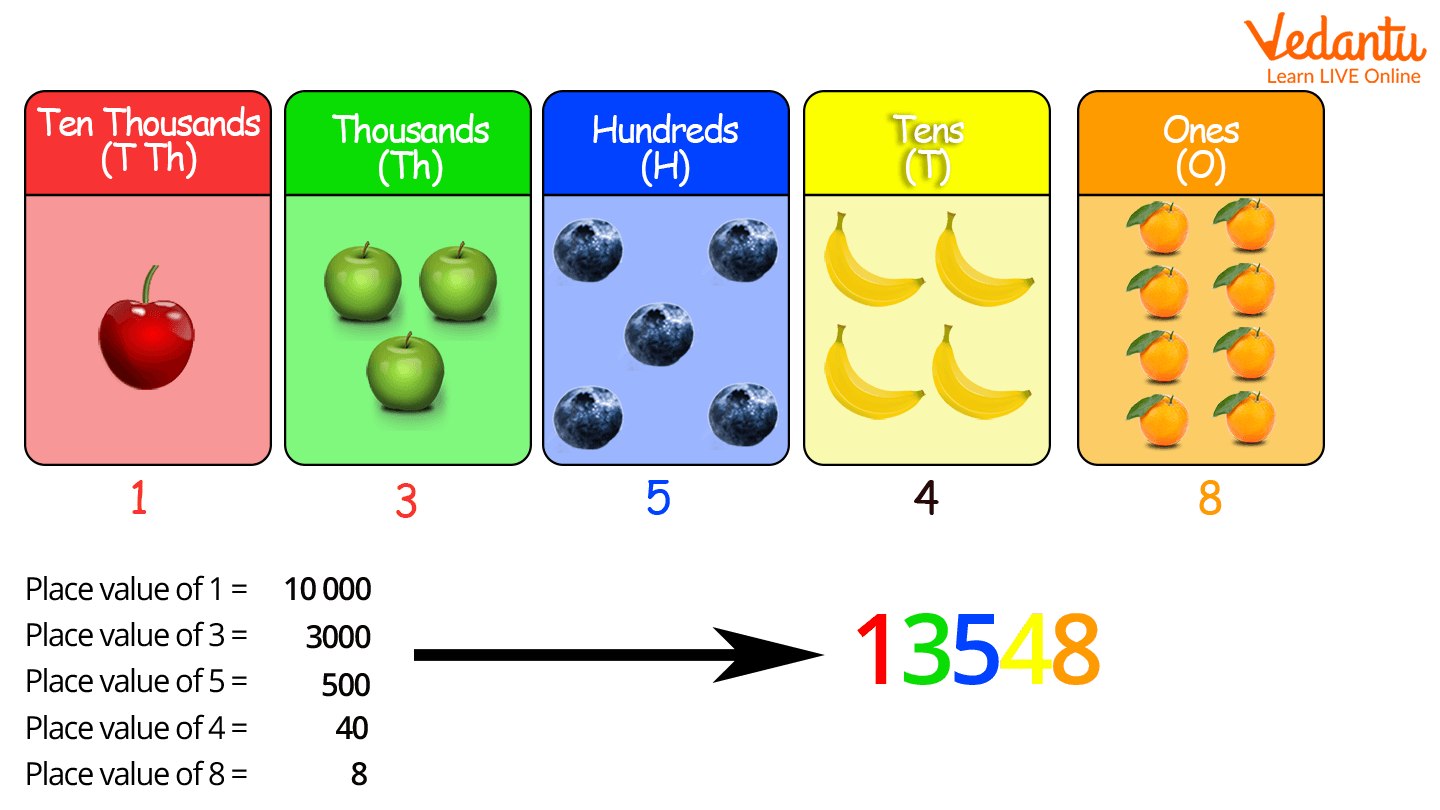• Number Line - It is a straight line used to represent numbers. The numbers are placed at equal intervals along its length. Zero is considered the origin, so the numbers increase from left to right on the number line and decrease from right to left.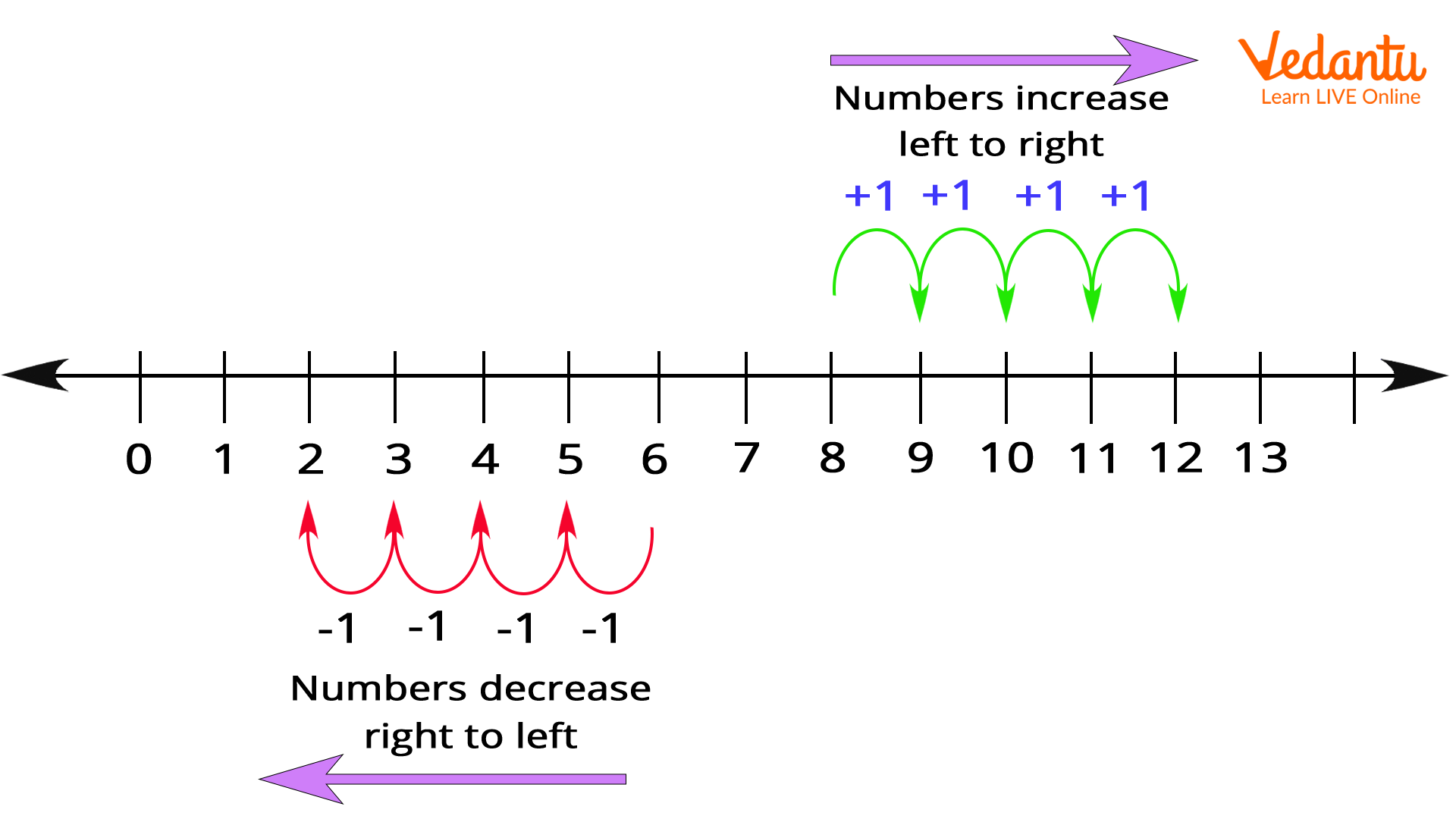### Counting and Algebra

• Unit - In maths, unit is used to refer to a number at the rightmost position or the one’s place. For example, in the number 9,87,635, the unit’s number is 5. While, in measurement a unit is the basic quantity used to measure different quantities. For example, the units of measuring length are millimetre, centimetre, metre, and kilometre.

• Decimal Point - It is a point used to separate the whole number part from the fractional part in a number.

## Geometry

• Angle - It is the conical figure formed by two lines meeting at a common endpoint. It is represented by the symbol ∠ and its value is measured in degrees.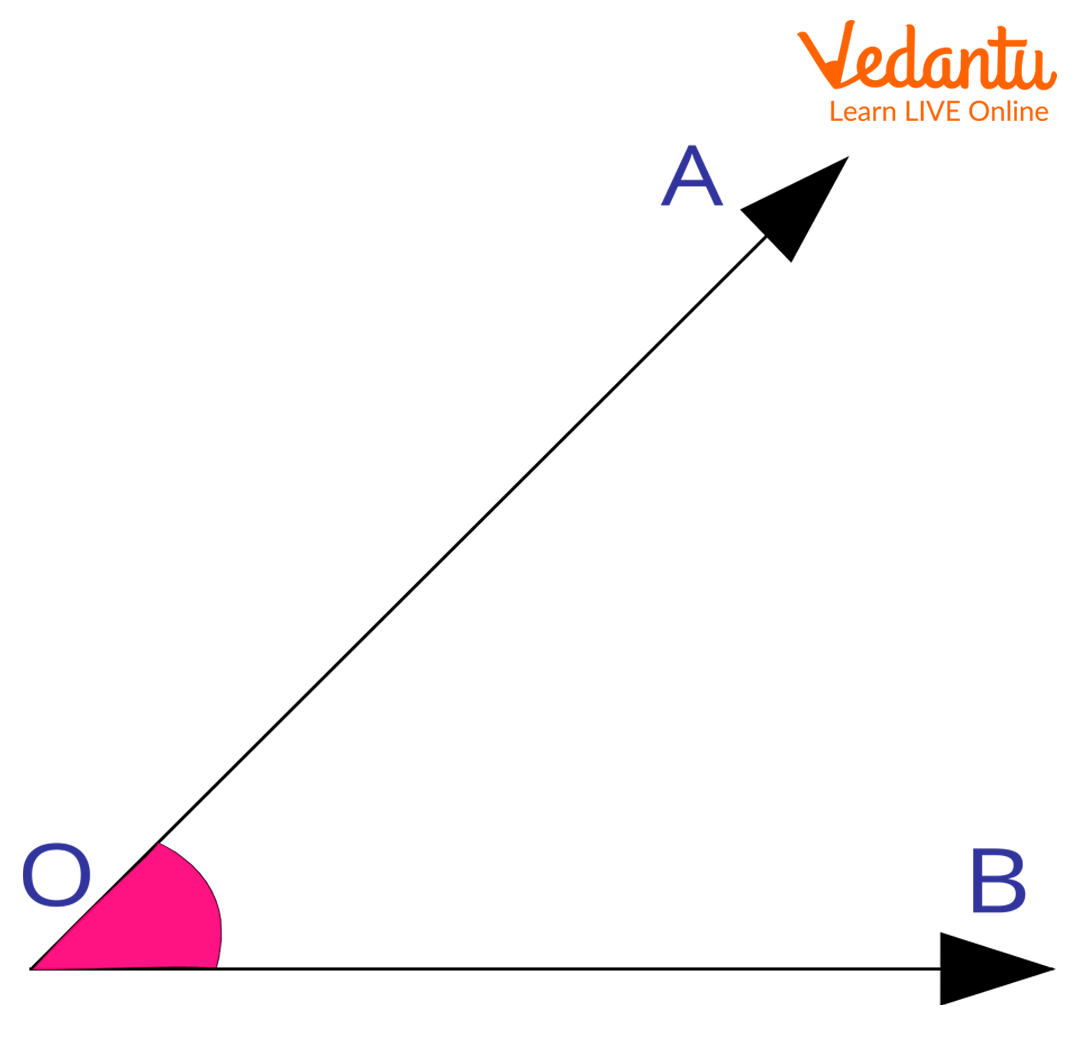• Two-Dimensional Shape (2-D) - It is a flat figure or a shape with only two dimensions – length and breadth. They do not have any thickness. For example, square, rectangle, circle, triangle, etc.

• Three-Dimensional Shape (3-D)- It is a flat figure or a shape with three dimensions – length, breadth, and height. For example, cube, cuboid, sphere, cone, cylinder, etc.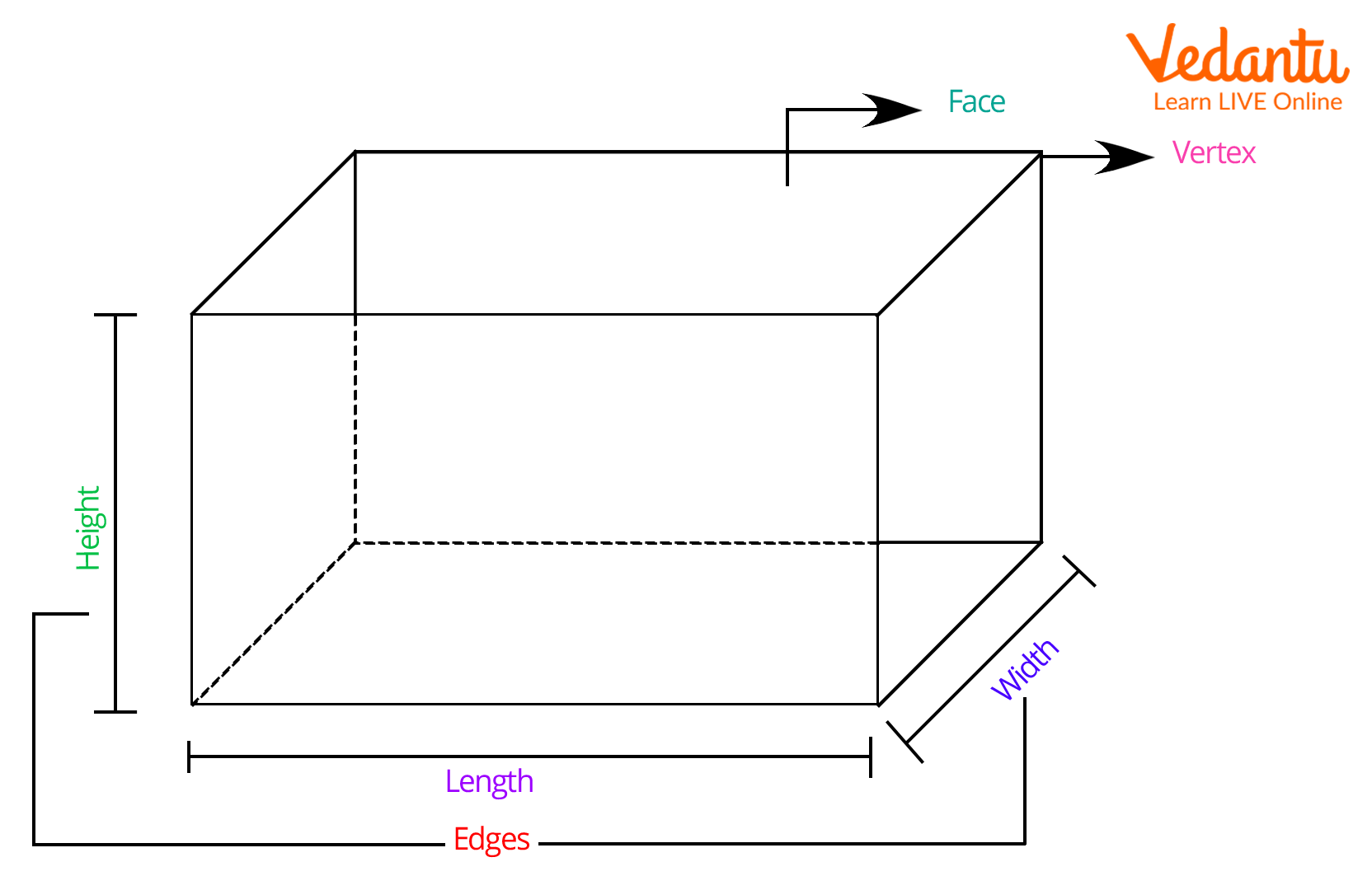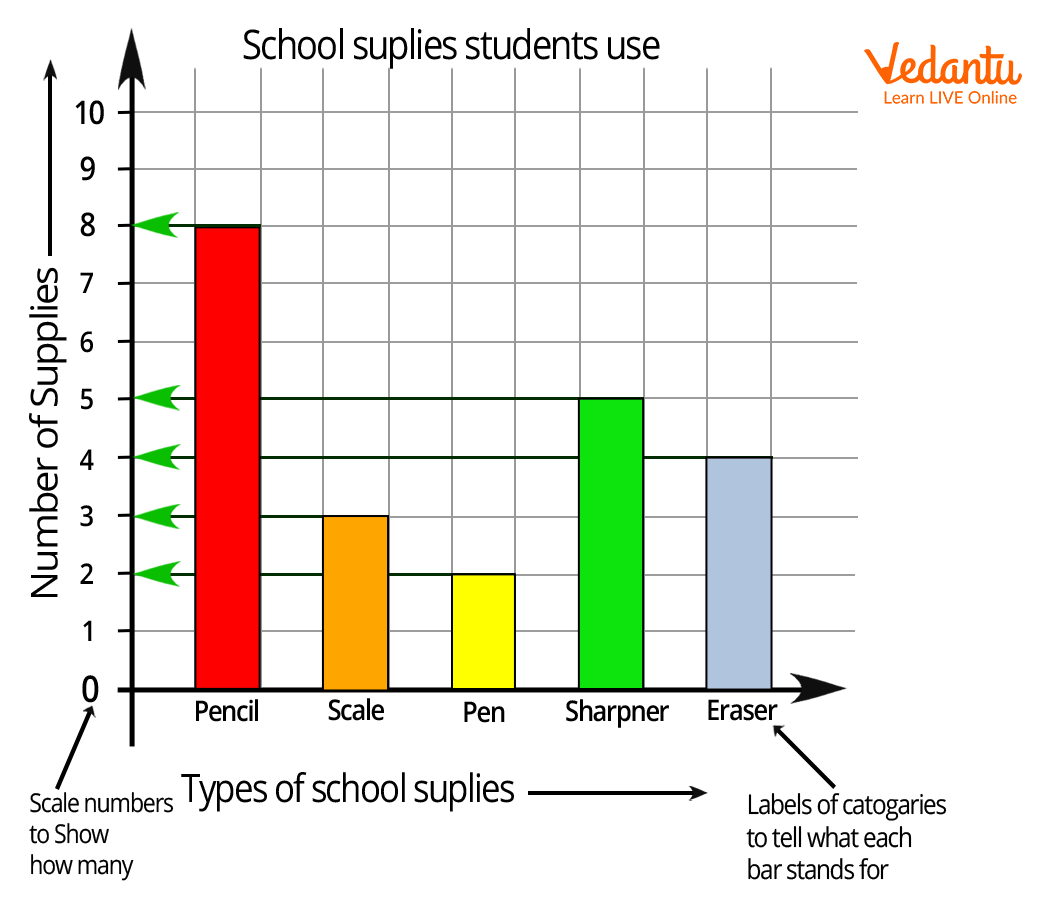## Conclusion

This maths glossary for second grade is not exhaustive. You can learn more such words starting with different letters and for different grades on our website. Learning maths vocabulary will give you an edge over your peers in the subject. So, happy learning!

Last updated date: 23rd Sep 2023
Total views: 136.2k
Views today: 2.36k

## FAQs on Maths Vocabulary for 2nd Grade Students

1. What is the importance of teaching maths vocabulary to second grade students?

A student should start learning new words no matter the grade level, however, teaching maths vocabulary according to the grade of the student is important. Starting with a basic maths glossary will enhance the students’ vocabulary and they will be better able to understand the subject.

2. What other maths topics should a second grader know?

Some important maths topics to teach students in second grade include number counting till 1000, addition and subtraction till 3-digits, learning the place value of three-digit numbers, being able to tell time, basic  bar graphs, basic word problems, etc.